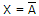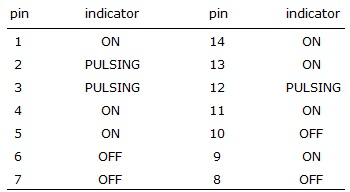# Digital Electronics - Logic Gates

### Exercise :: Logic Gates - General Questions

21.

What are the pin numbers of the outputs of the gates in a 7432 IC?

 A. 3, 6, 10, and 13 B. 1, 4, 10, and 13 C. 3, 6, 8, and 11 D. 1, 4, 8, and 11

Explanation:

No answer description available for this question. Let us discuss.

22.

The output of a NOT gate is HIGH when ________.

 A. the input is LOW B. the input is HIGH C. power is applied to the gate's IC D. power is removed from the gate's IC

Explanation:

No answer description available for this question. Let us discuss.

23.

If the input to a NOT gate is A and the output is X, then ________.

 A. X = A B.C. X = 0 D. none of the above

Explanation:

No answer description available for this question. Let us discuss.

24.

A logic probe is used to test the pins of a 7411 IC with the following results. Is there a problem with the chip and if so, what is the problem?A. Pin 6 should be ON. B. Pin 6 should be pulsing. C. Pin 8 should be ON. D. no problem

Explanation:

No answer description available for this question. Let us discuss.

25.

How many inputs of a four-input AND gate must be HIGH in order for the output of the logic gate to go HIGH?

 A. any one of the inputs B. any two of the inputs C. any three of the inputs D. all four inputs